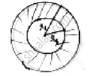Share

# The Area Enclosed Between the Concentric Circles is 770cm2. If the Radius of Outer Circle 21cm. Find the Radius of Inner Circle - CBSE Class 10 - Mathematics

ConceptAreas of Combinations of Plane Figures

#### Question

The area enclosed between the concentric circles is 770cm2. If the radius of outer circle 21cm. find the radius of inner circle

#### Solution

Radius of outer circle = 21𝑐𝑚Radius of inner circle = 𝑅2

Area between concentric circles = area of outer circle – area of inner circle

⇒ 770 =22/7(212 − R22)

⇒ 212 − 𝑅22 = 35 × 7 = 245

⇒ 441 – 245 = 𝑅22

⇒𝑅2= sqrt(196) = 14 𝑐𝑚

Radius of inner circle = 14cm.

Is there an error in this question or solution?

#### APPEARS IN

Solution The Area Enclosed Between the Concentric Circles is 770cm2. If the Radius of Outer Circle 21cm. Find the Radius of Inner Circle Concept: Areas of Combinations of Plane Figures.
S